## Position Space and Momentum Space

We can represent a state with either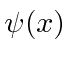or with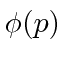. We can (Fourier) transform from one to the other.

We have the symmetric Fourier Transform.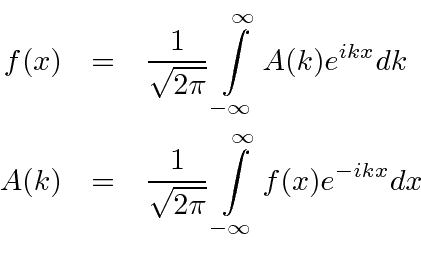When we change variable from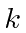to, we get the Fourier Transforms in terms ofand.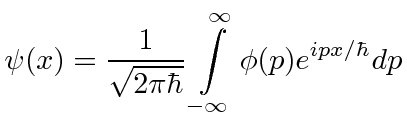These formulas are worth a little study. If we defineto be the state with definite momentum, (in position space) our formula for it isSimilarly, the state (in momentum space) with definite positionisThese states cannot be normalized to 1 but they do have a normalization convention which is satisfied due to the constant shown.

Our Fourier Transform can now be read to say that we add up states of definite momentum to getand we add up states of definite position to get.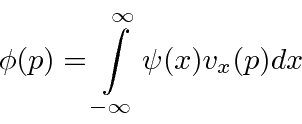There is a more abstract way to write these states. Using the notation of Dirac, the state with definite momentum,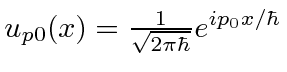might be written asand the state with definite position,might be writtenThe arbitrary state represented by eitheror, might be written simple as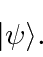The actual wave functionwould be written asThis gives us the amplitude to be atfor any value of.

We will find that there are other ways to represent Quantum states. This was a preview. We will spend more time on Dirac Bra-ket notation later.

Jim Branson 2013-04-22